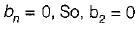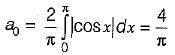Courses

# Continuous Time Fourier Series

## 8 Questions MCQ Test Topicwise Question Bank for GATE Electrical Engineering | Continuous Time Fourier Series

Description
This mock test of Continuous Time Fourier Series for GATE helps you for every GATE entrance exam. This contains 8 Multiple Choice Questions for GATE Continuous Time Fourier Series (mcq) to study with solutions a complete question bank. The solved questions answers in this Continuous Time Fourier Series quiz give you a good mix of easy questions and tough questions. GATE students definitely take this Continuous Time Fourier Series exercise for a better result in the exam. You can find other Continuous Time Fourier Series extra questions, long questions & short questions for GATE on EduRev as well by searching above.
QUESTION: 1

Solution:
QUESTION: 2

Solution:
QUESTION: 3

### A periodic triangular wave is shown in figure its fourier components will consists only of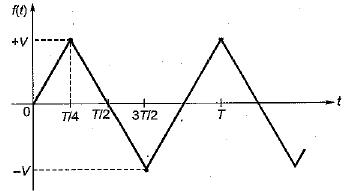Solution:
QUESTION: 4

Determine the Fourier series coefficient for given periodic signal x(t) is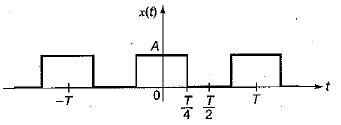Solution: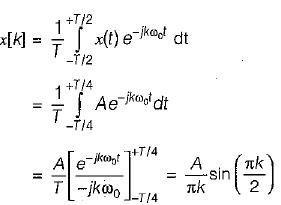QUESTION: 5

Consider the three continuous time signals with fundamental period of T = 1/2
x(t) = cos4πt
y(t) = sin 4πt
z(t) = x(t)·y(t)

The Fourier co-efficient of z(t) are given by

Solution: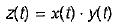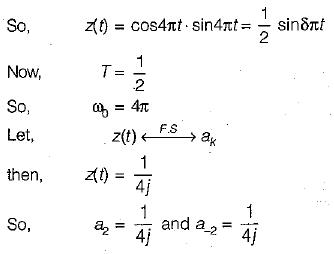QUESTION: 6

Consider a continuous time periodic signal x(t) given by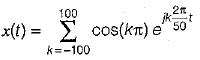Solution: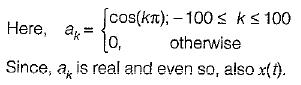QUESTION: 7

The Fourier series coefficient of time domain signal have been given. Determine the corresponding time domain signal and choose correct option.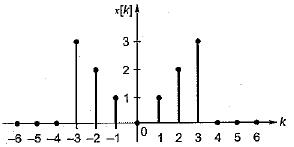Solution: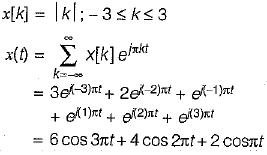QUESTION: 8

If f(x) = | cosx | ; (-π < x < π), then the fourier series coefficient a0 and b2 is

Solution: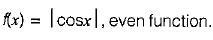∴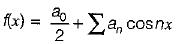Here